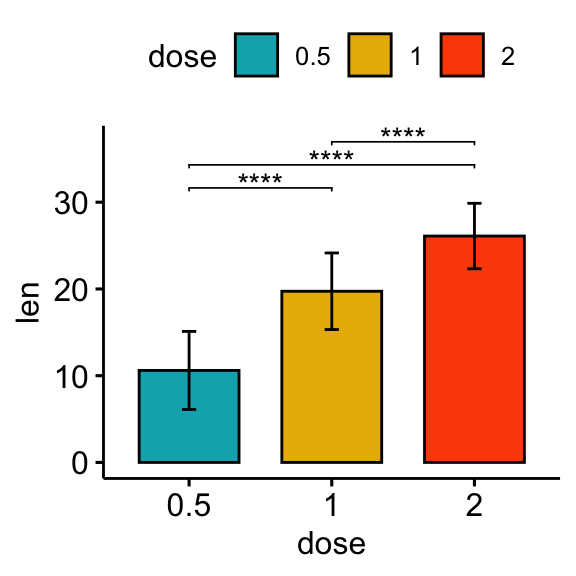# How to Add P-Values onto Horizontal GGPLOTS#### How to Add P-Values onto Horizontal GGPLOTS

This article describes how to add p-values onto horizontal ggplots using the R function stat_pvalue_manual() available in the ggpubr R package.

• Horizontal plots can be created using the function coord_flip() [in ggplot2 package].
• When adding the p-values to a horizontal ggplot, you need to specify the option coord.flip = TRUE in the function stat_pvalue_manual() [in ggpubr package]. The options vjust (vertical adjustment) and hjust (horizontal adjustment) can be also specified to adapt the position of the p-value labels.

Note that, in some situations, the p-value labels are partially hidden by the plot top border. In these cases, the ggplot2 function scale_y_continuous(expand = expansion(mult = c(0, 0.1))) can be used to add more spaces between labels and the plot top border. The option mult = c(0, 0.1) indicates that 0% and 10% spaces are respectively added at the bottom and the top of the plot.

Contents:

## Prerequisites

Make sure you have installed the following R packages:

• ggpubr for creating easily publication ready plots
• rstatix provides pipe-friendly R functions for easy statistical analyses.

library(ggpubr)
library(rstatix)

## Data preparation

# Transform dose into factor variable
df <- ToothGrowth
df$dose <- as.factor(df$dose)
head(df, 3)
##    len supp dose
## 1  4.2   VC  0.5
## 2 11.5   VC  0.5
## 3  7.3   VC  0.5

## Create simple plots

# Box plots
bxp <- ggboxplot(df, x = "dose", y = "len", fill = "dose",
palette = c("#00AFBB", "#E7B800", "#FC4E07"))

# Bar plots showing mean +/- SD
bp <- ggbarplot(df, x = "dose", y = "len", add = "mean_sd", fill = "dose",
palette = c("#00AFBB", "#E7B800", "#FC4E07"))

## Pairwise comparisons

### Statistical test

In the following example, we’ll perform T-test using the function t_test() [rstatix package]. It’s also possible to use the function wilcox_test().

stat.test <- df %>% t_test(len ~ dose)
stat.test
## # A tibble: 3 x 10
## * <chr> <chr>  <chr>  <int> <int>     <dbl> <dbl>    <dbl>    <dbl> <chr>
## 1 len   0.5    1         20    20     -6.48  38.0 1.27e- 7 2.54e- 7 ****
## 2 len   0.5    2         20    20    -11.8   36.9 4.40e-14 1.32e-13 ****
## 3 len   1      2         20    20     -4.90  37.1 1.91e- 5 1.91e- 5 ****

### Vertical plots with p-values

# Box plot
stat.test <- stat.test %>% add_xy_position(x = "dose")
bxp + stat_pvalue_manual(stat.test, label = "p.adj.signif", tip.length = 0.01)

# Bar plot
stat.test <- stat.test %>% add_xy_position(fun = "mean_sd", x = "dose")
bp + stat_pvalue_manual(stat.test, label = "p.adj.signif", tip.length = 0.01)### Horizontal plots with p-values

#### Using the adjusted p-value significance levels as labels

# Horizontal box plot with p-values
stat.test <- stat.test %>% add_xy_position(x = "dose")
bxp +
stat_pvalue_manual(
stat.test, label = "p.adj.signif", tip.length = 0.01,
coord.flip = TRUE
) +
coord_flip()

# Horizontal bar plot with p-values
stat.test <- stat.test %>% add_xy_position(fun = "mean_sd", x = "dose")
bp + stat_pvalue_manual(
stat.test, label = "p.adj.signif", tip.length = 0.01,
coord.flip = TRUE
) +
coord_flip()#### Using the adjusted p-values as labels

# Using p.adj as labels
stat.test <- stat.test %>% add_xy_position(x = "dose")
bxp +
stat_pvalue_manual(
stat.test, label = "p.adj", tip.length = 0.01,
coord.flip = TRUE
) +
coord_flip()# Change the orientation angle of the p-values
# Adjust the position of p-values using vjust and hjust
# Add more spaces between labels and the plot border
bxp +
stat_pvalue_manual(
stat.test, label = "p.adj", tip.length = 0.01,
coord.flip = TRUE, angle = 0,
hjust = 0, vjust = c(0, 1.2, 0)
) +
scale_y_continuous(expand = expansion(mult = c(0.05, 0.3))) +
coord_flip()## Comparisons against reference groups

### Statistical tests

stat.test <- df %>% t_test(len ~ dose, ref.group = "0.5")
stat.test
## # A tibble: 2 x 10
## * <chr> <chr>  <chr>  <int> <int>     <dbl> <dbl>    <dbl>    <dbl> <chr>
## 1 len   0.5    1         20    20     -6.48  38.0 1.27e- 7 1.27e- 7 ****
## 2 len   0.5    2         20    20    -11.8   36.9 4.40e-14 8.80e-14 ****

### Vertical plots with p-values

# Vertical box plot with p-values
stat.test <- stat.test %>% add_xy_position(x = "dose")
bxp +
stat_pvalue_manual(stat.test, label = "p.adj", tip.length = 0.01) +
scale_y_continuous(expand = expansion(mult = c(0.05, 0.1)))

# Vertical bar plot with p-values
stat.test <- stat.test %>% add_xy_position(fun = "mean_sd", x = "dose")
bp +
stat_pvalue_manual(stat.test, label = "p.adj.signif", tip.length = 0.01) +
scale_y_continuous(expand = expansion(mult = c(0.05, 0.1)))### Horizontal plots with p-values

# Horizontal box plot with p-values
stat.test <- stat.test %>% add_xy_position(x = "dose")
bxp +
stat_pvalue_manual(
stat.test, label = "p.adj", tip.length = 0.01,
coord.flip = TRUE
) +
scale_y_continuous(expand = expansion(mult = c(0.05, 0.1))) +
coord_flip()

# Horizontal bar plot with p-values
stat.test <- stat.test %>% add_xy_position(fun = "mean_sd", x = "dose")
bp +
stat_pvalue_manual(
stat.test, label = "p.adj", tip.length = 0.01,
coord.flip = TRUE
) +
scale_y_continuous(expand = expansion(mult = c(0.05, 0.1))) +
coord_flip()# Show p-values at x = "group2"
stat.test <- stat.test %>% add_xy_position(x = "dose")
bxp +
stat_pvalue_manual(
stat.test, label = "p = {p.adj}", tip.length = 0.01,
coord.flip = TRUE, x = "group2", hjust = 0.4
) +
scale_y_continuous(expand = expansion(mult = c(0.05, 0.2))) +
coord_flip()

# Make the p-values horizontal using the option angle
stat.test <- stat.test %>% add_xy_position(x = "dose")
bxp +
stat_pvalue_manual(
stat.test, label = "p = {p.adj}", tip.length = 0.01,
coord.flip = TRUE, x = "group2", angle = 90
) +
scale_y_continuous(expand = expansion(mult = c(0.05, 0.1))) +
scale_x_discrete(expand = expansion(mult = c(0.05, 0.3))) +
coord_flip()## Conclusion

This article describes how to add p-values onto horizontal ggplots using the R function stat_pvalue_manual() available in the ggpubr R package. See other related frequently asked questions: ggpubr FAQ.

•Thank you for another excellent guide.
I would like to ask if it is possible to show only the upper standard deviation line (+ sd) on the barplot (ggbarplot) chart.
Best wishes

•Kassambara

Thank you for the positive feedback, highly appreciated.

It’s possible to show only the upper error bars by specifying the option error.plot = "upper_errorbar":

library(ggpubr)
ggbarplot(
ToothGrowth, x = "dose", y = "len", add = "mean_sd",
error.plot = "upper_errorbar"
)
••